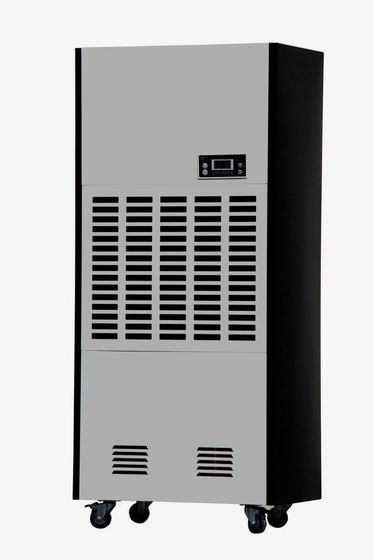### 技术交流technical

﻿### 工业除湿机设计计算书

.设计要求：

1. JB/T7769-954.2.1要求名义工况为：干球温度27 湿球温度21.2℃   RH=60%   大气压101.325kpa

2. 设计除湿量：8kg/h±5%

3. 设计使用温度18℃-32℃

4. 设计使用制冷剂为HCFC R22

5. 设计最大负荷工况为：干球温度32 湿球温度23

6. 设计低温工况为：干球温度18 湿球温度13.5

7. 设计使用三相380V 50HZ电源

8. 噪音

9. 蒸发温度5℃,过热度8K,冷凝温度48℃，过冷度2K。

1. 名义工况下的其它空气参数：

T=27℃  RH=60% 工况下水蒸气分压力：

Pq=RHⅹPqb=60%ⅹ3564.56=2138.7Pa

A工况下的空气密度

ρA=P0.003484/（273.15+t）=1.18KG/M3

2. 流程图

AB过程为减焓减湿过程，见焓混示意图A段到B段，设计使B点工况为：Tb=15.5℃ RHb=95%  d b=10.55 g/kg

Hb=42kJ/kg

BC点为等湿加温过程，C点的工况焓hc=HA+P /M(空气质量流量)

dC=10.55 g/kg

3. 制冷量及相关设备参数

=2298.2 m3

=0.753ⅹ（61.3ⅹ42）=14.5kW

23.38x1.1=25.7 m2

S迎面=F/3600x（2∽3）=2300/3600 /（2∽3）=0.32∽0.213取0.3

1. C点工况：在除湿制冷工况下，一般情况全封闭（涡旋）式压缩机制冷系数3∽3.5之间。

hc= hb+［（1+1/COP）P/M］

= hb+28=66kj/kg

hc= 1.005tc+dc(2500+1.84tc)X0.001得到

（1. 005+1.84tc）= hc-2500 dc

tc=（ hc-2500 dc）/[（1.005+1.84tc）X0.001]=41.42℃

RHc=XP/(0.622+X)Pqb(41.42)

=(9.46*0.001*101325)/[(0.622+9.46*0.0=01)*7904.2]

=19.2%

2.冷凝器面积：

t冷凝=（241.42-14）/[2.3lg（48-15.5）/（48-12）]=16.2

S=（1+1/COP）P/ △t冷凝*R冷凝

=（18.6*0.001）/（16.7*28）=39.7

39.7*1.2=48m2

A点热力学参数：hA=255 TA=42

B点热力学参数：hB=255 TA=42

C点热力学参数：hC=255 TA=42  比容VPC=44.6dm3/kg

ZHONGYOU上海众有CFZ-8工业除湿机﻿